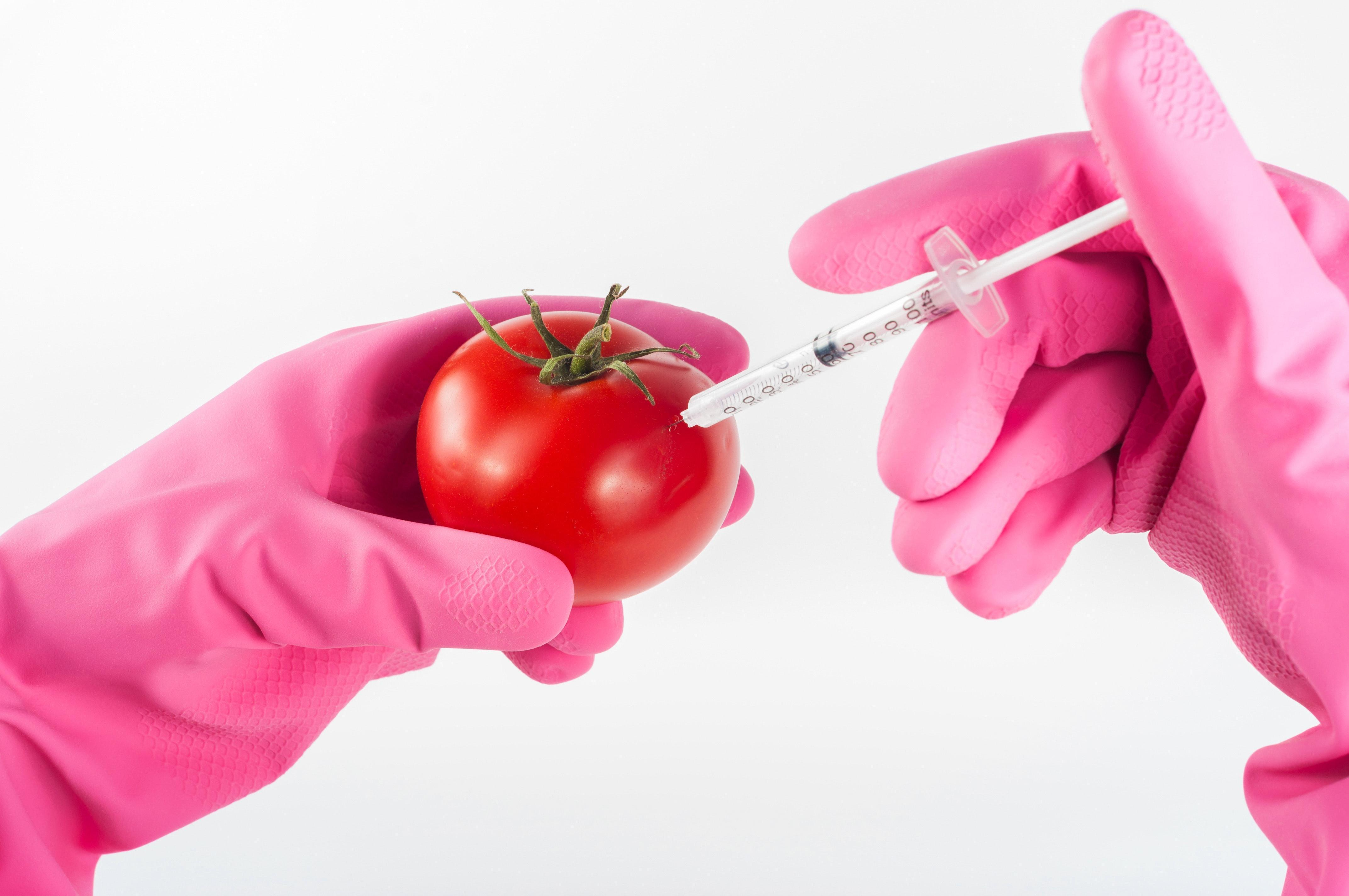# Genetic AlgorithmsThis Post is a general discussion on how genetic algorithms work and how to model them. Typically GAs are built to solve a single problem, however the concept of genetic improvement can be extended into building functionality too.. (this isn’t a topic of study so check out this site for context for that.)

During my studies I eve build a new python module to construct a nice framework for GAs.

Genetic Algorithms fall under a branch of machine learning called feature selection. Techniques of using genetic algorithms for generating solutions to problems typically revolve around heuristically improving members of a population who represent these solutions. The concepts of a genetic algorithm come from nature; like nature we create a survival of the fittest competition to evaluate a population then kill off the weakest members. After this cull we create offspring from the most successful population or introduce new members from a predefined source. This process is then repeated until we stop, or forever in the case of nature.

We say a genetic algorithm is structured in the following way. Given a population, $$P$$, each with unique genes, and a number of generations, $$G\in \mathbb{N}$$, the algorithm will create $$G$$ loops of scoring and potentially removing each of the members of the population. It does this by using a heuristic function $$f(p_i)\mapsto \mathbb{R},\ p_i \in P$$. This function, $$f$$, is defined beforehand in a way which describes the goal of our investigation. Defining a cutoff or bottleneck $$b<|P|$$, such that on conclusion of scoring the population, the top $$b$$ ranking members by score can be kept and the rest discarded. Once we have removed a certain percentage of our population we can rebuild it using a series of crossovers and mutations (and possibly introducing new members into the population).

• Crossovers take in 2 members of the population and return a new member based on some parameters of the 2 `parents’. For example, our crossover takes the first half of a sequence from one and the second half from the other, merging them to form the third.
• Mutations allow a (possibly targeted) change in a single member of the population. A mutation has 2 parameters, a potency $$M_p\in \mathbb{R}>0$$ and a frequency $$M_f\in [0,1]$$. $M_p$ describes how strong the mutation is, the higher it is the larger change to the member occurs. $$M_f$$ explains the percentage of how many members of the population are mutated.